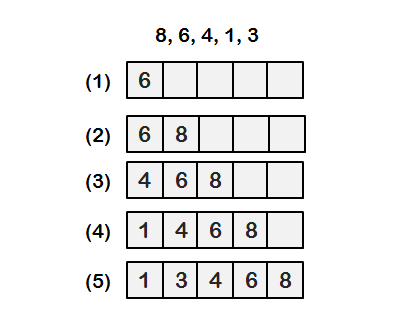# Insertion Sort Example In Data StructureNilanchala   |   #C Programming   |   Sep 17, 2023,

In this tutorial, we will see insertion sort example In data structure . An insertion sort is quite simple to understand and simple to implement.

1. An insertion sort visits each element of the array, in turn. As it visits a particular element, it scans the array from the beginning to end to determines where in that segment of the array the current value belongs.
2. It then inserts the current value in that location and shifts every element of the array to the right, up to the present location. It then goes on to the next location (value) in the array.
3. Notice that one index is going from 0 to n and for each such value and another index is scanning the array from 0 to the value of the first index. The result of this is – that this type of sort is O(n2). Insertion sort is slower.

## Case Study

This is a naturally occurring sorting method exemplified by a card player arranging the cards dealt to him. He picks up the cards as they are dealt and inserts them into the required position. Thus at every step, we insert an item into its proper place in an already ordered list.

Example: Sort the following list (8,6,4,1,3) using insertion sort## Insertion sort algorithm

Thus to find the correct position search the list till an item just greater than the target is found. Shift all the items from this point one, down the list. Insert the target in the vacated slot.

```INPUT: LIST[ ] of N items in random order.
OUTPUT: LIST[ ] of N items in sorted order.
1. BEGIN
2. FORI = 2 TO N DO
3. BEGIN
4. IF LIST[I] LIST[I-1]
5. THEN BEGIN
6. J = I.
7. T = LIST [I] /*STORE LIST [I] */
8. REPEAT /* MOVE OTHER ITEMS DOWN THE LIST.
9. J = J-1
10. LIST [J + 1] = LIST [J];
11. IF J = 1 THEN
12. FOUND = TRUE
13. UNTIL (FOUND = TRUE)
14. LIST [I] = T
15. END
16. END
17. END
```

## Example: Program to sort n numbers using insertion sort.

```#include<stdio.h>

void insertion_sort(int a[],int n)
{
int i,j,item;
for(i=0;i<n;i++)
{
/* item to be inserted */
item = a[i];
/* Try from (i-1)th position */
j=i-1;
while(j>=0 && item<a[j])
{
A[j+1] = a[j] /* Move the item to the next position */
j--; /* and update the position */
}
A[j+1]=item; /* appropriate position found and so insert item */
}
}

void main()
{
int i, n,a;
printf("Enter the no. of elements to sort n");
scanf("%d", &n);
printf("Enter n elements n");
for(i=0;i<n;i++)
scanf("%d",&a[i]);
insertion_sort(a,n);
printf("The sorted array is n");
for(i=0;i<n;i++)

printf("%dn",a[i]);

}
```### Nilanchala

I'm a blogger, educator and a full stack developer. Mainly focused on Java, Spring and Micro-service architecture. I love to learn, code, make and break things.

## Related articles2 months ago ·C Programming

### Selection Sort Example In Data Structure

EasyPost2 months ago ·C Programming

### Bubble Sort Example In Data Structure

EasyPost2 months ago ·C Programming

### Sorting Methods In Data Structures

EasyPost2 months ago ·C Programming

### Binary Search Algorithm In Data Structure

EasyPost2 months ago ·C Programming

### Sequential Search Algorithm in Data Structure

EasyPost2 months ago ·C Programming

EasyPost